# A Novel Calculation Model for Liquid Carrying Capacity of Low-Yield Gas Wells

A Novel Calculation Model for Liquid Carrying Capacity of Low-Yield Gas Wells
Yangtze University, Caidian District, Wuhan 430100, China

Key Laboratory of Production Engineering for Oil and Gas (Yangtze University), Wuhan 430100, China

The Fifth Oil Extraction Plant, PetroChina Changqing Oilfield Company, Xi’an 710000, China

Lukeqin Oil Extraction Plant, PetroChina Tuha Oilfield Company, Turpan 838206, China

Corresponding Author Email:
LiuJie@Yangtzeu.edu.cn
Page:
145-150
|
DOI:
https://doi.org/10.18280/ijht.380116
12 October 2019
|
Accepted:
27 December 2019
|
Published:
31 March 2020
| Citation

OPEN ACCESS

Abstract:

In low-yield gas wells, liquid loading is a common problem due to energy loss and pressure drop along the wellbore, especially in the middle to late phases of development. Because liquid loading is inevitable, it is necessary to calculate how much liquid can be unloaded under the current yield. Inspired by dimensionless analysis, this paper explores the mechanism of liquid carryover at low gas-liquid ratios, analyzes the dynamics of gas-liquid two-phase flow, and then establishes a calculation model of liquid carrying capacity for low-yield gas wells. The effectiveness of our model was verified through the analysis on actual low-yield gas wells. The results show that our model can calculate the liquid carrying capacity at a high accuracy (>85%). The research results provide a good reference for normal production in the late phase of gas fields.

Keywords:

1. Introduction

Liquid loading is a prominent issue in gas well production. Many experiments and mathematical models have been designed to solve this issue [1-7]. The minimum amount of gas required to fully carry the produced liquid to surface is known as the critical liquid carrying capacity. Through repeated improvements, the theory on critical liquid carrying capacity is now mature and comprehensive [8-11].

For high-yield gas wells, the liquid loading can be predicted easily in the early phase of development. With the elapse of time, the gas well energy gradually depletes, making it difficult to reach the critical liquid carrying capacity. In this case, produced liquid will inevitably accumulate in the wellbore, and the onsite management team should try to unload the liquid as much as possible.

In 2011, Liu et al.  analyzed the hydraulics of wellbore liquid loading, and created a calculation model for the maximum liquid carrying capacity, according to the multiphase flow theory and the momentum equation of the annular flow in the vertical column. However, their model only applies to high-yield gas wells with wellbore liquid in the state of annular mist flow, failing to adapt to liquid carrying capacity under low yield conditions.

To sum up, the previous research on liquid carrying capacity mainly focuses on the critical liquid carrying velocity, aiming to accurately predict liquid loading and time the drainage and gas recovery. Nevertheless, there is little report that quantifies the accumulated liquid. It is important to quantify the liquid volume and identify the flow pattern in the wellbore in the middle and late phases of development, because the yield in these phases falls below the critical liquid carrying velocity.

To make up for the gap, this paper carries out an indoor air carrying experiment with a small air volume, and investigates the liquid carrying capacity of the gas well and the flow pattern of the gas-liquid mixture in the wellbore. After that, a new calculation model was established to determine the liquid carrying capacity of low-yield gas wells based on the liquid carrying mechanism with low gas-liquid ratio and dimensionless analysis. The research results provide a reference for implementing drainage and gas recovery measures.

2. Air Carrying Experiment

2.1 Experimental device

The device for air carrying experiment is illustrated in Figure 1. The centerpiece is a small 2.7m-long tube (length: 2.7m; inner diameter: 30mm) that emulates the oil pipe and casing of the gas well. The tube is made of transparent plexiglass, making it easy to observe the flow patterns and liquid carrying conditions of the gas-liquid mixture. The tube is connected at the top to a gas-liquid separator (inner diameter: 201.3mm), which is also made of transparent plexiglass, and connected at the bottom to the inlet pipe.

The liquid level was controlled by a stainless-steel barrel with adjustable height, in order to simulate the low-yield conditions. The air inlet of the device is connected to a pressure gauge to simulate the downhole pressure, while the top of the separator is connected to a gas flow meter (precision: ±1%), which controls the gas flow to the level of a low-yield gas well. The test device is supported by a small oil-free air compressor (rated pressure: 0.8 MPa; storage: 230L) and a high-pressure self-priming vortex pump (maximum velocity: 8.5m3/h; maximum lift: 55m; self-priming height: 9m).

## 1.png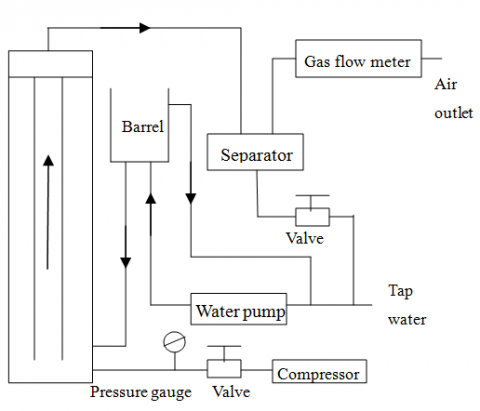Figure 1. The sketch map of experimental device

2.2 Methodology

In low-yield gas wells, the liquid often accumulates into a liquid column in the wellbore, exerting a back pressure. The back pressure impedes the liquid carryover. The taller the column, the greater is the back pressure. However, the tube adopted in our device is too small to simulate the high liquid level in actual gas wells. To solve the problem, a dimensionless height parameter H was introduced to simulate the field conditions. The parameter is defined as the ratio of the height of the gas column above the free liquid surface (hs) to the tube length.

The air carrying experiment was carried out at room temperature (25°C) under the pressure of 0.1MPa. The experimental media were the air and tap water. During the experiment, the stainless-steel barrel was adjusted to control the liquid level, and thus the gas volume above the liquid surface. The hs value was kept at 0.6, 1.17, 1.3, 1.4, 1.5, 1.6 and 1.7m in turn. The amount of intake air was gradually increased by adjusting the opening degree of the valve. Once the liquid was carried upward stably, the gas volume and liquid volume in the separator were recorded for further analysis. The flow pattern of the gas-liquid mixture was also observed in details. The gas-liquid velocity curves at different hs values are presented in Figure 2 below. Each curve was plotted separately to facilitate the analysis.

## 2.png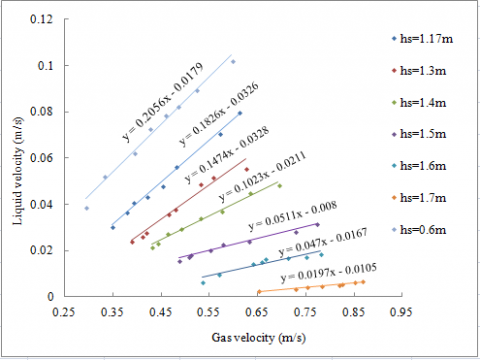Figure 2. The gas-liquid velocity curves at different hs values

As shown in Figure 2, the liquid velocity at different hs values increased at different rates with the growing gas velocity; the greater the hs value, the smaller the slope of the curve. These trends can be explained as follows: In the case of low yield, the gas cannot carry liquid continuously upward, and the wellbore mixture is a slug flow. The upward motion is mainly driven by the liquid slug that wraps the droplets against gravity and friction loss. The continued growth of gas volume gradually accelerates the frequency of the slug, pushing up the amount of liquid being carried over. As a result, the liquid velocity increases with the gas velocity. Nevertheless, the increase in the hs value pushes the liquid level away from the wellhead. Then, the mixture flow faces a greater potential energy and friction loss, i.e. more energy is required to carry out the liquid. That is why the liquid velocity growth slows down with the increase in the hs value.

After sorting out the experimental data, it is found that, under the same liquid level, the liquid carrying capacity increases with the gas volume; under the same gas volume, the liquid carrying capacity decreases with the increase in the hs value. Hence, the correlations of liquid carrying capacity with liquid level and gas volume were obtained intuitively for low-yield gas wells.

3. Calculation Model of Liquid Carrying Capacity

3.1 Liquid carrying mechanism

In actual gas production, both yield and liquid level change with time. Therefore, dimensionless analysis was carried out to establish a calculation model for liquid volume in low-yield gas wells, with the aim to quantify the effects of hs value and gas volume on liquid carrying capacity.

For a low-yield gas well, the gas has a limited liquid carrying capacity, and the liquid tends to accumulate in the wellbore. Over the time, the liquid phase becomes continuous, and the flow pattern changes from annular mist flow to slug flow (Figure 3). Then, separated sections of gas and gas-liquid mixture can be observed in the wellbore, due to the density and velocity differences between gas and liquid.

## 3.png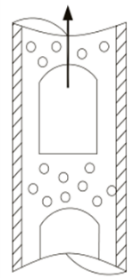Figure 3. The sketch map of the block flow

During gas production, the liquid slug moves upward to the wellhead to unload the liquid, under the combined action of the gas energy and the surface energy released by breakage of Taylor bubbles. Because the slug moves faster than the liquid film, the slug will merge with the liquid film in the upper part of the tube wall, forming a new upward-moving slug. In the meantime, the liquid at the end of the slug will partially fall off under gravity and friction [13-15], producing a liquid film on the tube wall. The merging and generating of liquid film reach a dynamic equilibrium. Under the push of gas, the liquid slug moves from the middle of the wellbore to the wellhead, and thus lifts liquid out of the wellbore. Because of the low yield, only a small volume of liquid is eventually carried over.

3.2 Model construction

It is very difficult to quantify the entrained liquid in the gas-liquid two-phase flow. To overcome the difficulty, the dimensionless analysis was combined with the kinetic theory for our research. It is assumed that the gas phase is incompressible in the gas-liquid two-phase flow. Hence, the equation of motion and continuity equation of gas could be derived from those of liquid. The equations of the two phases were coupled according to the stress equilibrium conditions at the gas-liquid interface, turning the liquid-bearing problem into the calculation of parameters of gas-liquid two-phase flow [16, 17]. Inspired by Liejin et al., the relationship between the parameters of each phase in the gas-liquid two-phase flow can be established as:

$Eu=Eu(\operatorname{Re},Fr,We,Ho,\frac{{{\rho }_{g}}}{{{\rho }_{\text{f}}}})$                (1)

where, $E u=(\Delta p) /\left(\rho f v_{s}^{2}\right)$ is Euler number (the ratio of pressure to inertial force); $\mathrm{Re}=\left(\rho_{f} v_{s} h_{s}\right) /(\mu f)$ is Reynolds number (the ratio of inertial force to viscous force); $F_{\mathrm{r}=}\left(v_{\mathrm{s}}^{2}\right) /\left(\mathrm{g} h_{\mathrm{s}}\right)$ is Froude number (the ratio of inertial force to gravity); $\mathrm{We}=\left(\left(\rho \mathrm{f-} \rho_{\mathrm{g}}\right) v_{\mathrm{s}}^{2}\right.$ $\left.h_{\mathrm{s}}\right) / \sigma$ is Weber number (the ratio of inertial force to surface tension); $H \mathrm{o}=\left(v_{\mathrm{s}} \tau\right) / h_{\mathrm{s}}$ is Harmonic hour (the ratio of spatial inertial force to instantaneous inertial force, generally fixed at 1).

In the spirit of dimensionless analysis, all numbers are determined by the nature of the fluid, except that the Euler number depends on velocity and pressure. This greatly eases the solving of the above equation.

Similarly, the relationship between the above numbers was adopted to solve the entrainment problem in low-yield gas wells. First, the entrainment coefficient Y was defined as:

$Y=\frac{\sum{{{M}_{\text{f}}}}}{{{M}_{\text{g}}}}$             (2)

where, the denominator is the mass velocity of the gas; the numerator is the total droplet entrainment per unit cross-sectional area per unit time. The Y value is exactly a dimensionless number. In this way, the entrainment coefficient could be combined with the kinetic parameters of the gas phase and the liquid phase, i.e. the solution of the entrainment coefficient could be converted into a phase relationship involving Reynolds number, Weber number, Froude number, etc.:

$Y\text{=}Y(\text{Re, Fr, We, Ho, }\frac{{{\rho }_{\text{g}}}}{{{\rho }_{\text{f}}}})$                   (3)

Considering the actual conditions and the previous results, the dimensionless parameters were combined to obtain the following equation:

$B=\frac{FR{{e}^{2}}}{We}$           (4)

Substituting Eq. (1) to Eq. (4), we have:

$B=\frac{v_{\text{g}}^{2}\rho _{\text{f}}^{2}\sigma }{\text{g}\mu _{\text{f}}^{2}({{\rho }_{\text{f}}}-{{\rho }_{\text{g}}})}$                  (5)

Then, Eq. (3) can be simplified as:

$Y=C{{B}^{m}}$               (6)

where, C and m are constants to be determined based on experimental data.

3.3 Parameter determination

The parameters C and m were fitted from the experimental data. For simplicity, the experimental data were preprocessed , and the dimensionless parameter H was introduced. Then, the correlations of H with C and m were established. Table 1 lists the values of C and m at different H values.

Table 1. The C and m values at different H values

 No. hs value [m] H value Coefficient C Exponent m 1 0.6 0.22222 123.5947 0.164 2 1.17 0.43333 78.52356 0.322 3 1.3 0.48148 51.28614 0.464 4 1.6 0.59259 7.48170 0.795 5 1.7 0.62963 0.97724 1.197

Based on the data in Table 1, the relationship between H and coefficient C and that between H and exponent m are plotted as Figure 4.

Parameter calculation shows that C and m both have a polynomial relationship with the H values, which agree well with the experimental data: C=-344.37H2-20.822H+145.99 and m=0.0508e4.7234H. It can be derived from Figure 4 that the coefficient C is negative when the H value is greater than 0.62, indicating that H value should fall between 0 and 0.62. This interval is also consistent with the experimental data.

Substituting Eqns. (2) and (5) into Eq. (6), we have:

${{M}_{\text{f}}}\text{=C}\times \frac{v_{\text{g}}^{2m}\rho _{\text{f}}^{2m}{{\sigma }^{m}}}{{{\text{g}}^{m}}\mu _{\text{f}}^{2m}{{({{\rho }_{\text{f}}}-{{\rho }_{\text{g}}})}^{m}}}\times {{M}_{\text{g}}}\times A\times t$          (7)

According to the original definitions and number of relationships, we have:

${{M}_{\text{g}}}={{\rho }_{\text{g}}}\times {{v}_{\text{g}}}$           (8)

${{M}_{\text{g}}}={{\rho }_{\text{g}}}\times {{v}_{\text{g}}}$               (9)

Substituting Eqns. (8) and (9) into Eq. (7), the liquid velocity can be obtained by:

${{v}_{\text{f}}}\text{=C}\times \frac{v_{\text{g}}^{2m+1}\rho _{\text{f}}^{2m-1}{{\rho }_{\text{g}}}{{\sigma }^{m}}}{{{\text{g}}^{m}}\mu _{\text{f}}^{2m}{{({{\rho }_{\text{f}}}-{{\rho }_{\text{g}}})}^{m}}}$           (10)

The above equation shows the relationship between the liquid velocity and various parameters in the gas-liquid two-phase flow, laying the basis for quantification of liquid carrying capacity. This is similar to the prediction method for critical liquid carrying capacity.

## 4a.jpg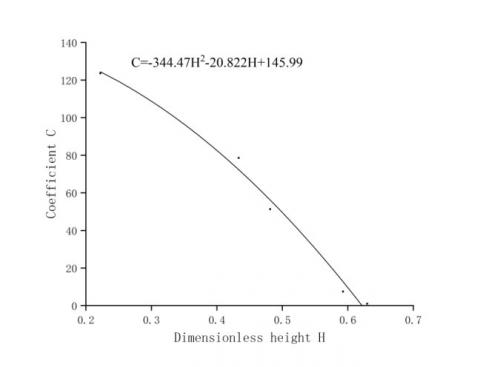(a) Curve between C and H

## 4b.jpg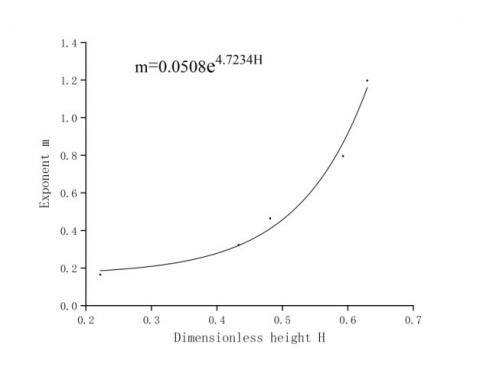(b) Curve between m and H

Figure 4. The relationship curves between H and undetermined parameters

3.4 Analysis of influencing factors

The liquid carrying capacity could be affected by multiple factors, ranging from gas type, tubing size, mining method, to well depth [19-22]. From Eq. (9), it can be seen that the calculation accuracy may vary greatly with the physical parameters (e.g. temperature and pressure) of the gas phase, which is assumed as a compressible fluid. The decrease in temperature will cause gas condensation along the wellbore. Since fluid has already accumulated and the low-yield gas wells are usually shallow, the temperature distribution of the wellbore can be illustrated by a linear curve. Meanwhile, the pressure change is a leading impactor, because the liquid phase in the wellbore is continuous [23-25]. Overall, the temperature change has a negligible impact on the liquid carrying capacity of low-yield gas wells, while the effect of pressure change must be considered.

Judging by our model, the velocity and density of the gas phase are directly involved in the calculation. Taking the H value of 0.3 for example, the overall impact factor M of the gas phase on liquid carrying capacity can be calculated by:

$M=\frac{v_{\text{g}}^{1.42}{{\rho }_{\text{g}}}}{{{({{\rho }_{\text{f}}}-{{\rho }_{\text{g}}})}^{0.21}}}$            (11)

As shown in Figure 5, the overall impact factor M decreased with the growing pressure of the gas phase, indicating that the calculated liquid velocity negatively correlate with gas phase pressure. In other words, fewer liquid is carried over by the gas, if the pressure of the gas phase decreases.

During normal gas production, the pressure of the gas-liquid mixture gradually reduces with the consumption and loss of various energies. The pressure peaks at the bottom of the well, reflecting that the liquid carrying capacity is the weakest under the conditions of the bottom hole. Therefore, the minimum liquid volume should be calculated based on the parameters of the well bottom.

## 5.jpg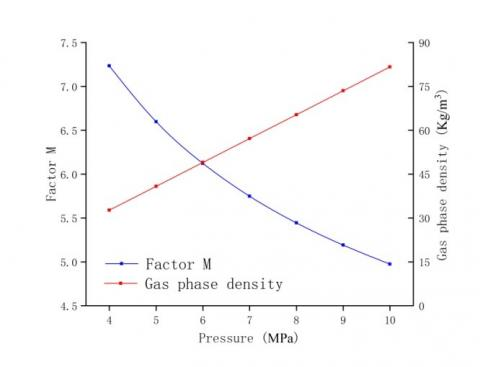Figure 5. The relationship between M and gas phase parameters

According to the equation of state, the temperature change was treated as a linear curve along the wellbore. Then, it is observed that the gas phase density decreases with the gas phase pressure. This means the wellhead has the smallest gas phase density, and the largest density difference between gas and liquid. Thus, the liquid carrying capacity is the strongest at the wellhead. The liquid carrying capacity must be calculated in the light of gas velocity. The significance of gas velocity is demonstrated by the minimum slippage loss and maximum energy consumption of the slug flow pattern in the wellbore under the low-yield production mode.

Table 2. The calculated liquid velocities at different H values

 No H value Coefficient C Exponent m Liquid velocity [m/s] 1 0.3 108.75 0.21 0.0624 2 0.4 82.56 0.34 0.0453 3 0.5 49.49 0.54 0.0375

According to Figure 4 and equation (10), it is clear that the liquid volume being carried over also depends on the hs value within the predefined interval. Table 2 lists the liquid velocities under different H values, with other parameters being constants. The results show that, as the H value increased in a certain range, the liquid velocity was reduced. This is because a high liquid column exerts a great back pressure, which weakens productivity and liquid carrying capacity. The findings are consistent with the theoretical results.

4. Example Analysis

To verify its effectiveness, our model was applied to calculate the liquid carrying capacity of 9 gas wells, whose gas flow is below the critical liquid capacity flow. The calculated results in Table 3 were basically the same as the field measurements, with an accuracy of more than 85%. Therefore, our model is feasible for computing liquid carrying capacity in actual gas fields.

Table 3. Calculation results of liquid carrying capacity in different productivity wells

 Well Depth [m] Oil pressure [MPa] Produced liquid [m3×d-1] Gas yield [104m3×d-1] Liquid volume [m3×d-1] State Error [%] Q-1 872.8 6.5 8.8000 1.0986 7.9959 Fluid 9.14 Q-2 1123.9 5.7 6.6462 0.7932 5.8352 Fluid 12.21 Q-3 1322.3 5.4 6.0000 0.8986 5.5575 Fluid 7.38 Q-4 996.5 5.0 8.9800 1.2096 7.5579 Fluid 13.84 Q-5 1266.3 8.5 11.2400 1.0670 12.262 Fluid 9.09 Q-6 1244.3 5.7 15.170 1.2058 13.2605 Fluid 12.59 Q-7 1260.5 4.9 9.2700 1.6364 10.84 Fluid 14.95 Q-8 1089.0 5.3 10.5800 1.0872 9.1261 Fluid 13.74 Q-9 1109.6 5.2 8.3000 0.8610 6.9730 Fluid 12.99 Mean error 11.77%

5. Conclusions

In the case of low yield, it is easy for liquid to accumulate in the wellbore, and the wellbore mixture is a slug flow. The upward motion is mainly driven by the liquid slug that wraps the droplets against gravity and friction loss. The droplets are partially carried up to the wellhead, and partially fall back to and accumulate at the bottom.

Inspired by dimensionless analysis, the dimensionless height parameter H was introduced to establish a calculation model for liquid carrying capacity of low-yield gas wells. The example analysis shows that the establish model can compute the liquid carrying capacity of actual gas wells at an accuracy greater than 85%.

The water carrying capacity peaks at the wellhead. The wellhead pressure is negatively correlated with the gas volume of the well. Hence, the wellhead pressure should be properly reduced to unload the liquid accumulated in the wellbore.

Future research will further improve the proposed model based on the field data from different regions.

Nomenclature

p = pressure, MPa;

vf = Apparent velocity of liquid, m/s;

vg = Apparent velocity of gas, m/s;

$\rho_{\mathrm{f}}$ = Gas density, kg/m3;

$\rho_{\mathrm{g}}$= Liquid density, kg/m3;

$\sigma$ = Gas-liquid interfacial tension, N/m;

hs = Air column height above the free surface, m;

$\sum M_{\mathrm{f}}$= Total droplet entrainment per unit cross-sectional area per unit time, kg/(m2×s);

Mg= Gas mass flow, kg/(m2×s);

A = Sectional area, m2;

t = time, s.

References

 Tan, Z., Lu, T., Liu, Y., Wu, L., Yang, Y. (2016). Technical ideas of recovery enhancement in the sulige gas field during the 13th five-year plan. Natural Gas Industry B, 3(3): 234-244. https://doi.org/10.1016/j.ngib.2016.05.008

 Waltrich, P.J., Posada, C., Martinez, J., Falcone, G., Barbosa, J.R. (2015). Experimental investigation on the prediction of liquid loading initiation in gas wells using a long vertical tube. Journal of Natural Gas Science and Engineering, 26: 1515-1529. https://doi.org/10.1016/j.jngse.2015.06.023

 Chen, D., Yao, Y., Fu, G., Meng, H., Xie, S. (2016). A new model for predicting liquid loading in deviated gas wells. Journal of Natural Gas Science and Engineering, 34: 178-184. https://doi.org/10.1016/j.jngse.2016.06.063

 Zhen, S., Yu, B., Li, D., Xu, F. (2013). Analysis of calculation of the horizontal gas well critical carrying liquid production in sulige gas field. Well Testing, 22(6): 21-23.

 Zhang, Z., Liao, R.Q., Fu, P., Su, Y.B., Luo, W., Zhang, D.X. (2018). Critical gas velocity prediction for vortex drainage gas wells, International Journal of Heat and Technology, 36(4): 1456-1462. https://doi.org/10.18280/ijht.360439

 Zhou, W., Li, T., Zhang, Y., Yi, J., Hu, G. (2015). Research on liquid level detection based on acoustic field characteristic of the oil wells. 2015 IEEE 10th Conference on Industrial Electronics and Applications (ICIEA). IEEE. https://doi.org/10.1109/ICIEA.2015.7334111

 Wang, X., Chen, P., Hu, D., Chen, Y. (2013). Dynamic simulation of gas well overflow based on drift-flux two-phase flow model. Journal of Southwest Petroleum University (Science & Technology Edition), 35(5): 118-122.

 Pitton, E., Ciandri, P., Margarone, M., Andreussi, P. (2014). An experimental study of stratified-dispersed flow in horizontal pipes. International Journal of Multiphase Flow, 67, 92-103. https://doi.org/10.1016/j.ijmultiphaseflow.2014.05.018

 Pan, J., Wang, W., Wei, Y., Chen, J., Wang L. (2018). A calculation model of critical liquid-carrying velocity of gas wells considering the influence of droplet shapes. Natural Gas Industry B, 5(4): 337343. https://doi.org/10.1016/j.ngib.2018.01.007

 Zhuo, C., Wu, X., Tang, J., Cen, X., Cao, M., Zhong, Z. (2016). Calculation of the critical liquid carry-over in the wellbore for downhole vortex dewatering gas recovery. Petroleum Geology & Oilfield Development in Daqing, 2016(6): 99-103.

 Chen, D., Yao, Y., Han, H., Fu, G., Song, T., Xie, S. (2016). A new prediction model for critical liquid-carrying flow rate of directional gas wells. Natural Gas Industry, 36(6): 40-44.

 Liu, J., Liao, R., Zhao, S. (2011). Quantitative analysis of the capability of liquid removing from different-productivity gas wells. Natural gas industry, 31(1): 62-64. https://doi.org/10.3787/j.issn.1000-0976.2011.01.013

 Lin, M.S., Liu, Y.H., Hu, Y.F., Che, D.F. (2013). Influence of the gas and liquid superficial velocity on slug frequency. AIP Conference Proceedings, 1547(1): 253-263. https://doi.org/10.1063/1.4816874

 Pedersen, S., Durdevic, P., Yang, Z. (2016). Challenges in slug modeling and control for offshore oil and gas productions: a review study. International Journal of Multiphase Flow, 88: 270-284. https://doi.org/10.1016/j.ijmultiphaseflow.2016.07.018

 Li, X., Zhang, P., Li, J.L., Wang, W.W., Chen, G.H. (2019). Analysis of deformation and internal flow patterns for rising single bubbles in different liquids. Chinese Journal of Chemical Engineering, 27(4): 745-758. https://doi.org/10.1016/j.cjche.2018.08.023

 Islam, M.R., Muzemder, A.T.M.S.H., Khan, A.I., Hira, M.A. (2016). Rate transient analysis of well-07 and well-10 of Habiganj gas field, Bangladesh. Journal of Petroleum Exploration & Production Technology, 7(2): 569-588. https://doi.org/10.1007/s13202-016-0278-y

 Zhao, J., Wang, B., Sum, A.K. (2017). Dynamics of hydrate formation and deposition under pseudo multiphase flow. Transport Phenomena and Fluid Mechanics, 63(9). https://doi.org/10.1002/aic.15722

 Xu, L.M., Li, M.J. (2020). Research on data and processing methods in dynamic comprehensive evaluation. Chinese Management Science, 28(1): 162-169.

 Peng, L.S., Qiao, L., Gong, M., Lü, Y.M. (2014). Factors affecting the production performance of coalbed methane wells with multiple-zone. Meitan Xuebao/Journal of the China Coal Society, 39(10): 2060-2067. https://doi.org/10.13225/j.cnki.jccs.2014.0541

 Chen, D.C., Yao, Y., Han, H., Fu, G., Song, T.J., Xie, S.X. (2016). A new prediction model for critical liquid-carrying flow rate of directional gas wells. Natural Gas Industry, 36(6): 40-44. https://doi.org/10.3787/j.issn.1000-0976.2016.06.006

 Zhang, X.D., Zhao, J.P., Zhang, S. (2014). Study on main control factors of gas drainage in coalbed methane well of tunliu minefield. Coal Science and Technology, 6.

 Shi, S.W., Du, J.J., Kang, D.Y., Chen, X.J., Qu, C.T., Yu, T. (2018). Study on fracturing flowback fluid treatment technology for shale gas in Yangzhou. IOP Conference Series: Earth and Environmental Science. https://doi.org/10.1088/1755-1315/121/5/052002

 Zhang, S.F., Ma, T.H., Cui, C.S., Zu, J. (2013). Research on temperature compensation of dynamic pressure measurement of oil and gas well. Electro-Optic Technology Application, 28(6): 87-90. https://doi.org10.3969/j.issn.1673-1255.2013.06.020

 Li, M.B., Liu, G.H., Li, J., Wei, X.Q., Gao, H.J. (2014). Research on wellbore temperature field with helical flow of non-Newtonian fluids in drilling operation. Petroleum Drilling Techniques (5): 74-79. https://doi.org10.11911/syztjs.201405013

 Xu, J., Hu, J., Wu, Z., Wang, S., Qi, B. (2013). Prediction of temperature and pressure distribution in HTHP injection gas wells with thermal effect of wellbore. Liquid Fuels Technology, 31(14): 1423-1438. https://doi.org/10.1080/10916466.2010.545785